Electron. J. Diff. Eqns., Vol. 2008(2008), No. 94, pp. 1-5.

### A characterization of dichotomy in terms of boundedness of solutions for some Cauchy problems Akbar Zada

Abstract:
We prove that a quadratic matrix of orderhaving complex entries is dichotomic (i.e. its spectrum does not intersect the imaginary axis) if and only if there exists a projection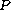on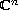such that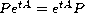for all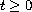and for each real number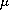and each vector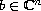the solutions of the following two Cauchy problems are bounded: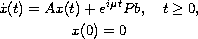and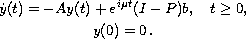Submitted May 29, 2008. Published July 05, 2008.
Math Subject Classifications: 47D06, 35B35.
Key Words: Stable and dichotomic matrices; Cauchy problem; spectral decomposition theorem.

Show me the PDF file (183 KB), TEX file, and other files for this article.Akbar Zada Government College University Abdus Salam School of Mathematical Sciences, (ASSMS) Lahore, Pakistan email: zadababo@yahoo.com